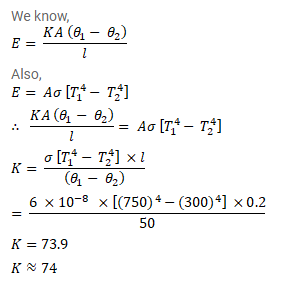# One end of a rod of length 20cm is inserted in a furnace at 800K.Question:

One end of a rod of length $20 \mathrm{~cm}$ is inserted in a furnace at $800 \mathrm{~K}$. The sides of the rod are covered with an insulating material and the other end emits radiation like a blackbody. The temperature of this end is $750 \mathrm{~K}$ in the steady state. The temperature of the surrounding air is $300 \mathrm{~K}$. Assuming radiation to be the only important mode of energy transfer between the surrounding and the open end of the rod, find the thermal conductivity of the rod. Stefan constant $\sigma=6.0 \times 10^{-8}{\mathrm{~W} / \mathrm{m}^{2}-K^{4}}^{4}$.

Solution: